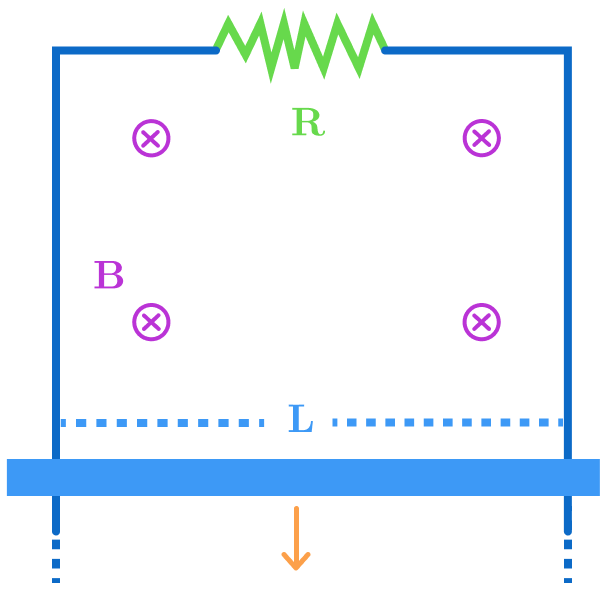# Mechanics or what?A horizontal conducting rod of mass $m$ is free to slide on two vertical conducting rails as shown. What is the terminal speed of the rod?

Details and Assumptions:

• A uniform magnetic field of intensity $B$ exists throughout the region and points into the screen.
• The length of the rod is $L$.
• The resistor has resistance $R$.

Bonus What will happen if the magnetic field is outward? Will the rod attain terminal velocity?

Try my next problem here

×